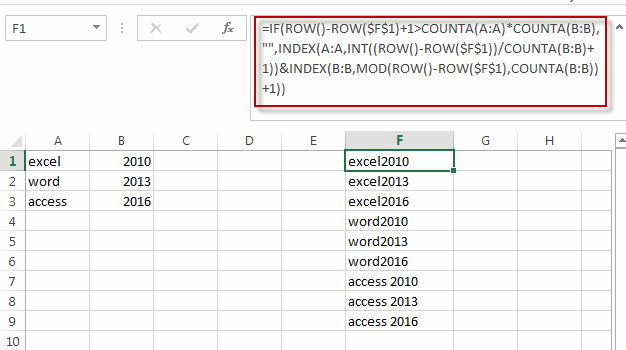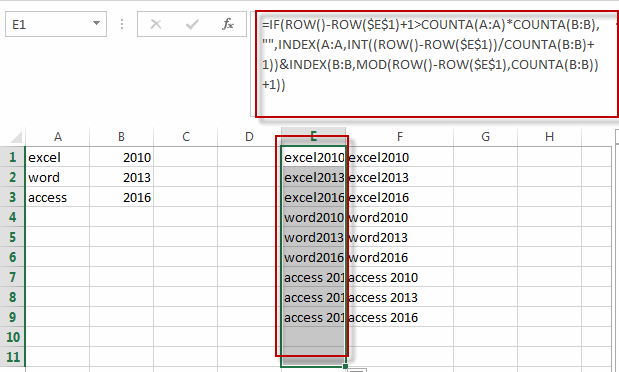# Generate All Possible Combinations of Two Lists in Excel

This post will guide you how to generate all possible combinations with a formula in excel. How do I create list with all possible combinations of two lists in Excel. How to list all combinations from two separate list or range in Excel.

## Generate All Possible Combinations of Two Lists in Excel

Assuming that you have two list of data in different column, A and B, and you want to get a list of all possible combinations from those two list in Column A and B. How to achieve it.

You can use a formula based on the IF function, the ROW function, the COUNTA function, The INDEX function and the MOD function. Like this:

`=IF(ROW()-ROW(\$F\$1)+1>COUNTA(A:A)*COUNTA(B:B),"",INDEX(A:A,INT((ROW()-ROW(\$F\$1))/COUNTA(B:B)+1))&INDEX(B:B,MOD(ROW()-ROW(\$F\$1),COUNTA(B:B))+1))`

Type this formula into Cell F1, and then drag the AutoFill Handle down column F until you get cells that look empty.Note: if you want to use this formula into other cell, you need to change the absolute cell reference to that cell. Such as: form F1 to E1.

`=IF(ROW()-ROW(\$E\$1)+1>COUNTA(A:A)*COUNTA(B:B),"",INDEX(A:A,INT((ROW()-ROW(\$E\$1))/COUNTA(B:B)+1))&INDEX(B:B,MOD(ROW()-ROW(\$E\$1),COUNTA(B:B))+1))`### Related Functions

• Excel INDEX function
The Excel INDEX function returns a value from a table based on the index (row number and column number)The INDEX function is a build-in function in Microsoft Excel and it is categorized as a Lookup and Reference Function.The syntax of the INDEX function is as below:= INDEX (array, row_num,[column_num])…
• Excel ROW function
The Excel ROW function returns the row number of a cell reference.The ROW function is a build-in function in Microsoft Excel and it is categorized as a Lookup and Reference Function.The syntax of the ROW function is as below:= ROW ([reference])….
• Excel IF function
The Excel IF function perform a logical test to return one value if the condition is TRUE and return another value if the condition is FALSE. The IF function is a build-in function in Microsoft Excel and it is categorized as a Logical Function.The syntax of the IF function is as below:= IF (condition, [true_value], [false_value])….
• Excel MOD function
he Excel MOD function returns the remainder of two numbers after division. So you can use the MOD function to get the remainder after a number is divided by a divisor in Excel. The syntax of the MOD function is as below:=MOD (number, divisor)….
• Excel INT function
The Excel INT function returns the integer portion of a given number. And it will rounds a given number down to the nearest integer.The syntax of the INT function is as below:= INT (number)…
• Excel COUNTA function
The Excel COUNTA function counts the number of cells that are not empty in a range. The syntax of the COUNTA function is as below:= COUNTA(value1, [value2],…)…

Related Posts

Average the Last N Numeric Values in Excel

AVERAGE function is one of the most popular functions in Excel. Apply AVERAGE together with some other functions, we can calculate average simply for some complex situations. In this article, we will introduce you to calculate average of the last ...

How To Sum the Largest N Values in Excel

Sometimes we may want to sum the largest N numbers or top N numbers in a range. In this article, we will show you the method of “SUM the Largest N Numbers” by a simple formula which consist of SUMPRODUCT ...

How to Sum the Smallest N Values in Excel

Sometimes we may want to sum the first smallest N numbers in a range in Excel. In this article, we will show you the method of “SUM the Smallest N Numbers” by a simple formula which consist of SUMPRODUCT and ...

How to Auto Fill Weekdays or Weekends in Excel

Sometimes we may want to enter a sequence of days in excel for some purpose, and we can press Ctrl+; to insert current date into cell, and then drag the cell down to attach following days into other cells, then ...

How to Create Dynamical Drop-Down List and Sort by Alphabetical Order in Excel

In our daily work we may need to create a dynamical dropdown list and sort all values by alphabetical order. To create a dropdown list like this, we need to apply some built-in features like ‘Define Name’ and ‘Data Validation’ ...

How to Highlight Every Other Row or Every Nth Row in Excel?

Sometimes we may want to highlight every other row or every Nth row in a spreadsheet to make data in different rows looks more clearly. Though we can apply table styles to highlight table rows automatically, we can also highlight ...

How to Count Duplicate Values Only Once in A Range in Excel?

When counting the number of times for objects appear in a list or a range, we usually record the duplicate value only once and ignore the redundant ones. We cannot apply formula with only one function to implement this in ...

How to Create Dynamic Drop Down List without Blank in Excel

This post will guide you how to create dynamic drop down list without blank cells in Microsoft Excel. In Excel, and you can use Data Validation feature to improve the efficiency of data entry in excel, and it also be ...

How to Stack Data from Multiple Columns into One Column in Excel

In previous article, I have shown you the method to split data from one long column to multiple columns by VBA and Index function. This time if we want to stack data from multiple columns to one column, how can ...

How to Count Only Unique Values Excluding Duplicates in Excel

We enter a list of numbers or products and there are some duplicates in the list, if we want to just do count for the unique values and exclude the duplicates, how can we do? Now you can follow the ...

Sidebar